# Introduction to Sorting Algorithms: Bubble Sort, Selection Sort and Insertion Sort

Input: An array X[] of n integers

Output: A permutation (reordering) of input such that X <= X <= X .... <= X[n-2] <= X[n-1]

Sorting is one of the fundamental problems in algorithms and data structure. But the critical question is - why do we study the design and analysis of the sorting algorithms? Here are some critical reasons:

• Various problem-solving approaches can be best understood using sorting algorithms: incremental approach (selection and insertion sort), divide and conquer (merge and quicksort), two pointers (merging and partition), problem-solving using a data structure (heap sort), etc.
• Sorting algorithms are best to understand the time and space complexity analysis of recursive and iterative codes.
• Sometimes we inherently need sorted data in our applications. For example, contact numbers are organized in sorted order of name in mobile phones.
• Sometimes organising the data into sorted order can help us to solve coding problems efficiently. In other words, sorting is also a famous problem-solving approach in programming. Similarly, several algorithms use sorting as a subroutine for their solution. For example, an algorithm for "finding the closest pair of points" and "finding convex hull" uses sorting for the pre-preprocessing of input.

This blog will discuss three O(n^2) iterative sorting algorithms: Bubble sort, Selection sort, and Insertion sort. Let's start and discuss one by one!

### Bubble sort algorithm

Bubble sort is a simple and inefficient sorting algorithm. It is generally one of the basic algorithms taught in programming courses to develop an excellent intuition about the working of algorithms. Sometimes It is also referred to as a "Sinking sort"! In 2007, former Google CEO Eric Schmidt asked presidential candidate Barack Obama during an interview about the best way to sort one million integers; Obama paused for a moment and replied, "I think the bubble sort would be the wrong way to go!" :)

Bubble sort idea

If we look at the element in the sorted array: the largest element is at the (n-1)th index, 2nd largest is at (n-2)th index, and so on. So a basic idea would be to first traverse the array from 0 to n-1 and place the largest element at the (n-1)th index. Again traverse the array from 0 to n-2 and place the second largest element at the (n-2)th index and...so on.

• After the end of 1st iteration, the largest element in the array bubbles up towards the (n-1)th index. Similarly, after the 2nd iteration, the 2nd largest element in the array bubbles up towards the (n-2)th index. and so on...
• In general, at ith iteration, we place the ith maximum element at (n - i)th index. This process will go on till the whole array is sorted.

We hope you got the idea! But now the critical question is: how do we implement and simulate the above process? Let's think!

Bubble sort steps

We can run two nested loops:

• At each stage of the outer loop, we aim to place one input value to its correct position in the sorted output. So we run the outer loop n times from i = 0 to n-1.
• After the first k iteration (i = 0 to k-1) of the outer loop, all k maximum elements have been placed from an index (n - k) to (n-1). So at the (k + 1)th iteration of the outer loop (where i = k), we need to place (k + 1)th maximum element at (n - k -1)th index. So we need to run an inner loop from n - k - 1 or n - i - 1 times. Or in simple words, the inner loop will run from j = 0 to n - i - 2. (Think)

``````for(int i = 0; i < n; i = i + 1)
{
for(int j = 0; j < n - i - 1; j = j + 1)
{
........
}
}
``````
• Now the critical question is: What would be the logic inside the inner loop? Idea would be simple: Starting from the first index, continuously compare adjacent pairs pairs of elements from left (j= 0) to right (j = n - i - 2), if they exist in wrong order then swap their positions. In such a way, larger elements “bubble up” to the right index n - i - 1.

``````for(int j = 0; j < n - i - 1; j = j + 1)
{
if(X[j] > X[j+1])
{
temp = A[j]
X[j] = X[j+1]
X[j+1] = temp
}
}
``````

Bubble sort visualisation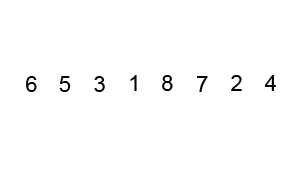Here is another visualisation: data was plotted in a coordinate system, with each point (xy) indicating that the value y is stored at index x. Then the data would be sorted by bubble sort according to every pixel's value.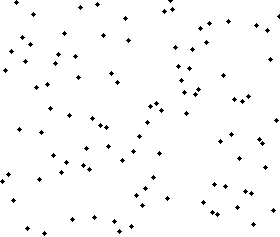Visualization Reference: Wikipedia

Bubble sort pseudocode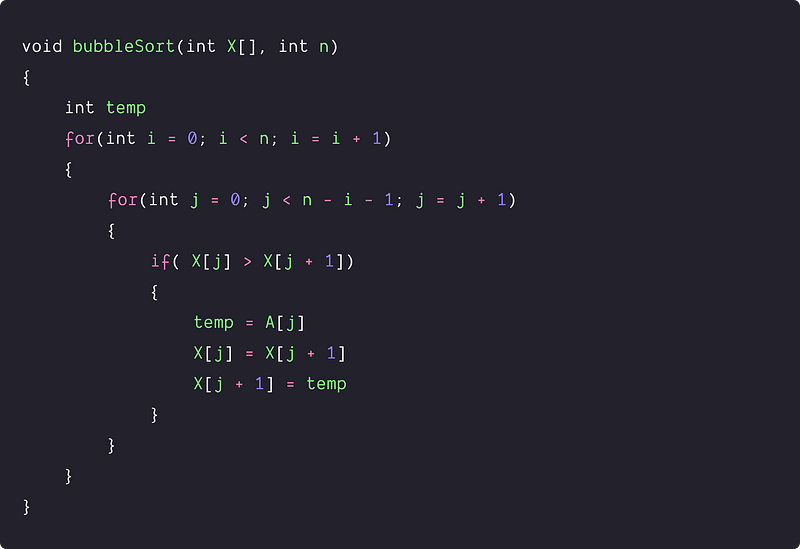Bubble sort complexity analysis

We are running two nested loops where comparison and swapping are the key operations. Regardless of the input, comparison operations are going to execute every time. In other size, the execution of the swap statement will depend upon the input, i.e., swapping happens only when comparison if( X[j] > X[j+1]) is true. So the comparison is the critical operation that decides the time complexity in the worst case.

For ith iteration of the outer loop, the inner loop will run (n-i-1) times. So count of loop operations = Sum of the simple arithmetic series (n-i-1) from i = 0 to n-1 = (n-1) + (n-2) + . . . + 2 + 1 = n(n-1)/2 = O(n^2)

Case 1: Worst-case scenario

When the input is reversely sorted, the comparison if( X[j] > X[j+1]) becomes true every time, and the swap operation will get executed every time. So, a reverse sorted array is a worst-case input for bubble sort.

• Count of comparison operations = Count of loop operations = O(n^2)
• Count of swap operations = Count of comparison operations = O(n^2)
• Time complexity in the worst case = O(n^2) + O(n^2) = O(n^2)

Case 2: Best-case scenario

When the input is already sorted, then comparison if( X[j] > X[j+1]) becomes false every time, and swap operation will not get executed. So, a sorted array is the best case input for bubble sort.

• Count of comparison operations = Count of loop operations = O(n^2)
• Count of swapping operations = O(1) (Think)
• Time complexity in the best case = O(n^2)

So in the both worst and best case, bubble sort run in O(n^2) time complexity. We are using constant extra space, so space complexity = O(1). Now the critical question is - can we optimize the

### Optimised bubble sort

Even if the array is sorted, the above algorithm always runs O(n^2) times. From another perspective: if the array gets sorted in the middle of the nested loop, there is no mechanism to stop the loop in the code, and the loop will continue to run unnecessarily. So, we can use this insight to optimize the bubble sort further. The idea would be to stop the algorithm if the inner loop didn't cause any swap! But the critical question is: how do we do it? Let's think!

If no element gets swapped inside the inner loop, it means array is sorted, and we can stop the bubble sort algorithms. We can detect such a stage of the algorithm using a boolean variable element_swapped inside the inner loop.

• Before starting any inner loop, we declare the flag element_swapped FALSE.
• When any swap happens inside the inner loop, we set this flag TRUE.
• By the end of the inner loop, if element_swapped is still FALSE, then it means no swap happens inside the inner loop, and the given array is already sorted, and we can break the process.
• If element_swapped is still TRUE, then we should go to the next iteration of the outer loop.

Optimised bubble sort pseudocode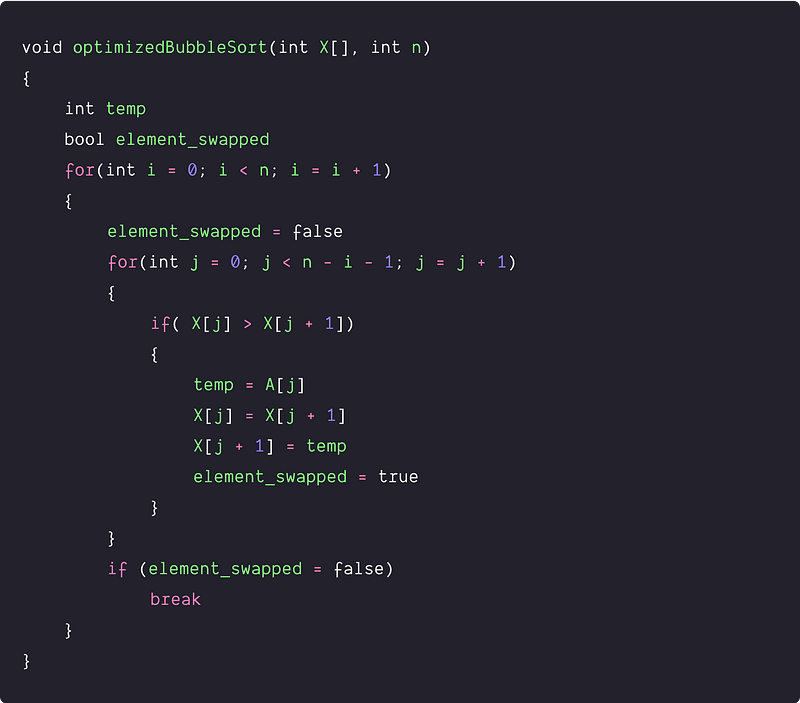In the above implementation, O(n) is the best-case running time for the bubble sort. If an array is already sorted, then bubble sort makes no swaps, and the algorithm can terminate after a single pass. (Think!) But there is no improvement in worst-case time complexity which is still O(n^2) in case of reverse sorted array.

Critical ideas to think!

• What would be the average case time complexity of the bubble sort?
• What is the idea of "stability" in case of sorting algorithms? is bubble sort stable sorting algorithm?
• The above pseudo-code sort the array in increasing order. How would we modify the above pseudo-code to sort the elements in decreasing order?
• How do we implement the bubble sort recursively?
• How can we sort linked lists and strings using bubble sort?
• Verify the correctness of above pseudo code at both boundaries: i = 0 and i = n-2.

### Selection sort algorithm

The idea of this algorithm is simple: we scan the whole array to find the smallest element. Once we find it, we swap the smallest element with the first element of the array. Then we look for the smallest element in the remaining array (without the first element) and swap it with the second element. This process goes on till the whole array gets sorted. In general, at any ith step of the algorithm, it maintains two subarrays in a given array.

1. The subarray X[0…i-1], which is already sorted
2. The remaining subarray X[i…n-1] is unsorted.

We build the partial solution or sorted array incrementally by finding the min value from the unsorted part and adding it to the sorted part. In every step of the iteration, our sorted part size will grow by one, and the unsorted part size will decrease by one. Now the critical question is: How do we implement the above idea? Let's think!

Selection sort steps

We need two nested loops: an outer loop to to place all n element in the sorted order and for every ith iteration of the outer loop, we run an inner loop to find the smallest element in the remaining array.

• We run outer loop from i = 0 to n-2 and repeat the following steps until the array is sorted. We also initialize the variable min_Index to stores the minimum value index of the unsorted part.
• Now for every index i, we run an inner loop from j = i to n-1 and find the index of the minimum value in the unsorted part.

``````int min_Index = i
for (int j = i; j < n; j = j + 1)
{
if (X[j] > X[min_Index])
min_Index = j
}
``````
• Now place the minimum value at ith index by swapping value at i with the value at min_Index i.e. swap(X[min_index], X[i])

Selection sort visualisation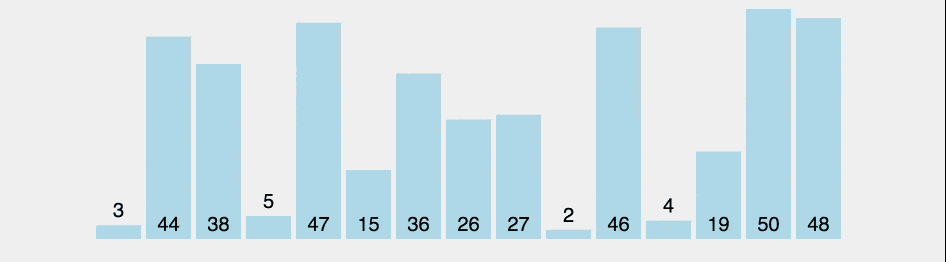Selection sort pseudocode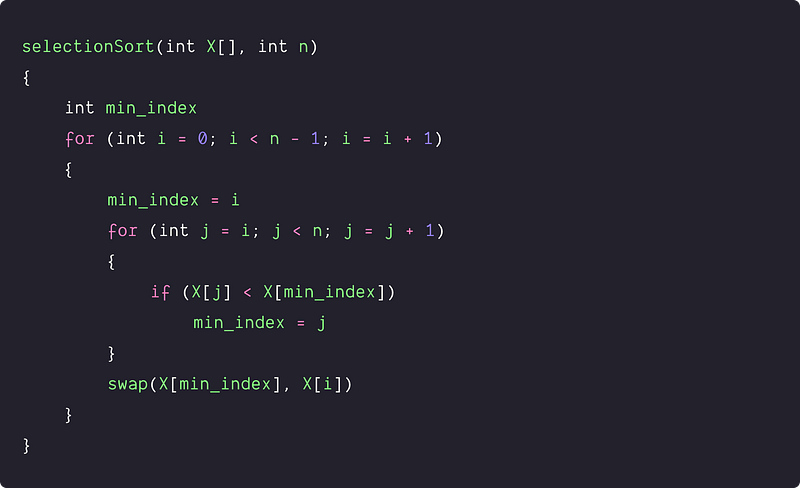Selection sort complexity analysis

We are running two nested loops where comparison, swapping and update of the min_index are the key operations. Regardless of the input, comparison and swapping operations are going to execute every time, but update of the min_index depend on the order of the input.

For every ith iteration of the outer loop, the inner loop will run (n-i) times. So count of loop operations = Sum of the simple arithmetic series of (n-i) from i = 0 to n-1 = (n) + (n-1) + (n-2) + . . . + 2 + 1 = n(n+1)/2 = O(n^2)

Case 1: Worst-case scenario

When the input is reversely sorted, then comparison if (X[j] < X[min_index]) becomes true every time, and the value of min_index will get updated every time (Think!). So, a reverse sorted array is a worst-case input for selection sort.

• Count of comparison operations = Count of loop operations = O(n^2)
• Count of swap operations = One swap on each iteration of the outer loop = n*O(1) = O(n)
• Total update operation of the min_index = Count of loop operations = O(n^2)
• Time complexity in the worst case = O(n^2) +O(n) + O(n^2) = O(n^2)

Case 2: Best-case scenario

When the input is already sorted, then comparison if (X[j] < X[min_index]) becomes false every time, and the value of min_index will not get updated every time (Think!). So, a reverse sorted array is a best-case input for selection sort.

• Count of comparison operations = Count of loop operations = O(n^2)
• Count of swap operations = One swap on each iteration of the outer loop = n*O(1) = O(n)
• Total update operation of the min_index = O(1)
• Time complexity in the worst case = O(n^2) +O(n) + O(1) = O(n^2)

So in the both worst and best case, selection sort run in O(n^2) time complexity. We are using constant extra space, so space complexity = O(1). Now the critical question is - can we optimize the

Critical ideas to think!

• What would be the average case time complexity of the selection sort?
• is selection sort a stable sorting algorithm?
• The above pseudo-code sort the array in increasing order. How would you modify the above pseudo-code to sort the elements in decreasing order?
• How do we implement the selection sort recursively?
• How can we sort linked lists and strings using selection sort?
• Verify the correctness of above pseudo code at both boundaries: i = 0 and i = n-2. Why are we running outer loop n-2 times? Why does the inner loop start from j = i?

### Insertion sort algorithm

It works the way you sort a hand of playing cards:

• We start with an empty left hand and all the remaining cards down on the table.
• Then we remove one card [key] at a time from the table [unsorted array], and insert it into the correct position in the left hand [sorted array].
• To find the correct position for the card, we compare it with each of the cards already in the hand, from right to left.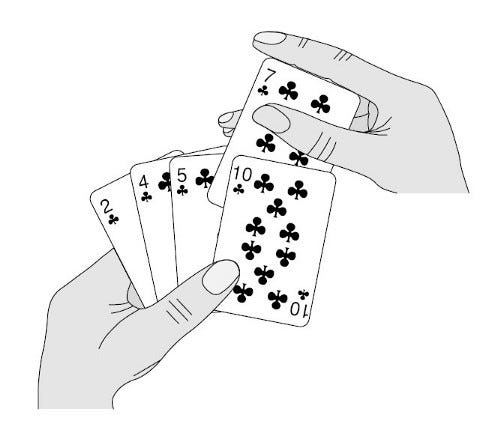Image Source: Algorithm by CLRS

Now the core idea is: If the first few elements of an array are sorted, we can easily insert an element at the proper place in the sorted part. At any ith step of the algorithm, it maintains two subarrays in a given array.

1. The subarray X[0…i-1], which is already sorted
2. The remaining subarray X[i…n-1] is unsorted.

We build the partially sorted array incrementally at each step of the iteration by choosing the first value from the unsorted part, i.e., X[i], and insert it into the sorted part X[0…i-1]. In every iteration step, our sorted part size will grow by one, and the unsorted part size will decrease by one. Now the critical question is: How we implement this idea? Let's think!

Insertion sort steps

We run a loop from i = 1 to n -1 and repeat the following steps until the whole array is sorted. We are starting from i = 1 because i = 0 is a case of a single element that is already sorted.

• We initialize a variable key with X[i]. We will place this value in the correct position of the partially sorted array X[0…i-1]
• For every index i, we run an inner while loop from j = i-1 to 0 and find the correct index to insert the value key in the partially sorted array X[0…i-1]. For this, We move elements of X[0…i-1] that are greater than the key to one position ahead of their current position to make space to insert the value key.

``````int key = X[i]
int j = i - 1
while (j >= 0 && X[j] > key)
{
X[j + 1] = X[j]
j = j - 1
}
``````
• After the inner while loop, j + 1 will be the correct position of the key in the partially sorted array. Insert key at (j+1)th index i.e. X[j+1] = key (Think!)
• Now move to the next element by incrementing the value of i.
• After the completion of the outer loop, array X[] contains the sorted output.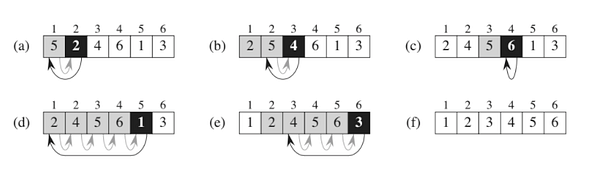Image Source — CLRS

Insertion sort pseudocode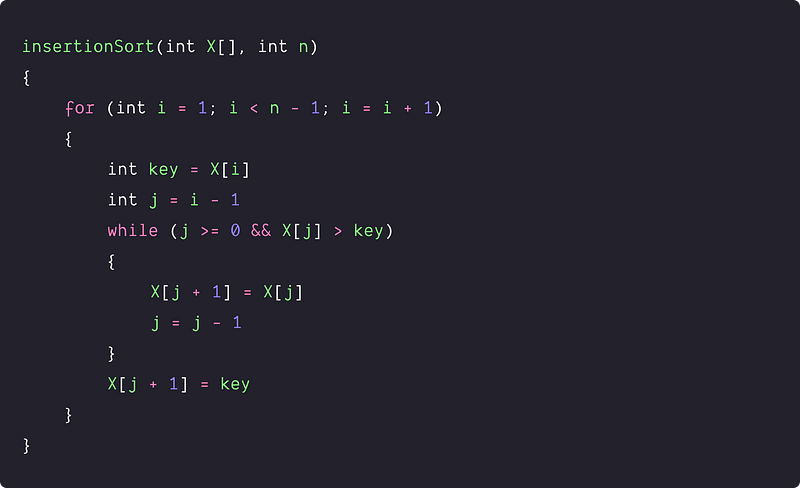Insertion sort complexity analysis

Best-case: Case of an already sorted array

In the case of a sorted array, the outer loop runs n-1 times, and the inner loop runs only one time. Why? because our input array is already sorted, and every time we enter into the while loop, the condition A[j] > key will be false, and we come out of the inner loop. In another way, the ith value is already there in the correct position, and we are only making one comparison at each iteration of the outer loop.

• Total comparison operation = n - 1 = O(n)
• Total shifting operation = 0 = O(1)
• Best case time complexity = O(n) + O(1) = O(n)

Worst-case: Case of a reverse sorted array

In reverse sorted array, outer loops run n-1 times, and for every iteration of outer loops, inner loops run i times. Why? Because our input array is reverse sorted and every iteration of the outer loop, the condition A[j] > key will be true for every value from j= i -1 to 0. Think!

In another way, we insert the ith value on its correct position and shift all the values from j = i -1 to 0 to the one place left. So at any ith iteration of the outer loop: count of comparison operation = i, count of shifting operation = i

• Total comparison operations = 1 + 2 + 3 + ….+n-2 + n-1 = n(n-1)/2 = O(n²)
• Total shifting operations = 1 + 2 + 3 + ….+n-2 + n-1 = n(n-1)/2 = O(n²)
• So worst-case time complexity = O(n²) + O(n²) = O(n²)

Critical ideas to think!

• Insertion sort is an efficient sorting algorithm for small input sizes. Why?
• How can we optimize the insertion sort further using binary search?
• Insertion sort is efficient than selection and bubble sort.
• It is efficient for the partially or almost sorted input data, i.e., the time complexity is O(kn), where each input element is no more than k places away from its sorted position. Think!
• Insertion sort is a stable sorting algorithm
• How do we calculate the average case time complexity of the Insertion sort?
• The above pseudo-code sort the array in increasing order. How we modify the above pseudo-code to sort the elements in decreasing order?
• How do we implement the Insertion sort recursively?
• How can we sort linked lists and strings using selection sort?
• Verify the correctness of above pseudo-code at both boundaries: i = 0 and i = n-2. Why are we running outer loop n-2 times? Why does the inner loop start from j = i?

### CritIcal concepts to explore further

• Concept of Stability in sorting algorithms
• What is comparison-based sorting? Explore the design, code, and analysis of Merge sort, Quicksort, Heap sort, Shell sort, BST sort
• Efficiency comparison of different sorting algorithms
• Linear time sorting algorithms: Counting sort, Radix sort, Bucket sort
• Searching in a sorted array: Binary search, Exponential Search
• Problem-solving using Sorting
• Use of sorting in real-life applications

### Sorting based coding problems to practice

• Sort an array of 0s, 1s, and 2s, Sort an array in a waveform
• Sort an array according to the order defined by another array
• Sort the even positioned elements in the ascending order and the odd positioned elements in the descending order.
• Count swaps required to sort an array using Insertion Sort
• Merge two sorted arrays, find the intersection of two sorted array
• Binary Insertion Sort, Recursive Insertion Sort

### Content references

• Algorithms by CLRS
• The Algorithm Design Manual by Steven Skiena

Enjoy learning, Enjoy coding, Enjoy algorithms!

Subscribe to get well-designed content on data structures and algorithms, machine learning, system design, oops, and mathematics. enjoy learning!

### We Welcome Doubts and Feedback!